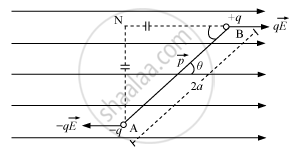Advertisement Remove all ads

# Derive the Expression for the Torque Acting on an Electric Dipole, When It is Held in a Uniform Electric Field. Identify the Orientation of the Dipole in the Electric Field, in Which It - Physics

Advertisement Remove all ads
Advertisement Remove all ads
Advertisement Remove all ads
Answer in Brief

Answer the following question.
Derive the expression for the torque acting on an electric dipole, when it is held in a uniform electric field. identify the orientation of the dipole in the electric field, in which it attains a stable equilibrium.

Advertisement Remove all ads

#### Solution

Dipole in a Uniform External FieldConsider an electric dipole consisting of charges − q and + q and of length 2a placed in a uniform electric field vec"E"  making an angle θ with the electric field.

Force on charge - q at "A" = -q vec"E" ("opposite to"_ vec"E")

Force on charge + q at "B" = qvec"E" ("along"_ vec"E")

The Electric dipole is under the action of two equal and unlike parallel forces, which give rise to a torque on the dipole.
T = Force x Perpendicular distance between the two forces

T = qE (AN) = qE (2a sin θ)

T = q(2a) E sinθ

T = pE sinθ

∴ vec"t" = vec"p" xx vec"E"

in a uniform electric field, the net force on dipole will always be zero but torque is zero for theta = 0° and theta = 180°

Now Potential Energy of a dipole in a uniform external electric field is given by the expression "P"."E" = - vec"p" .vec"E"

1. For theta = 0°, U = - pE  (minimum), the equilibrium will be stable and if the dipole is slightly displaced, it performs oscillations.
2. For theta = 180°, U = +pE(maximum), it will be an unstable equillibrium.

Concept: Dipole in a Uniform External Field
Is there an error in this question or solution?

#### APPEARS IN

Advertisement Remove all ads
Share
Notifications

View all notifications

Forgot password?